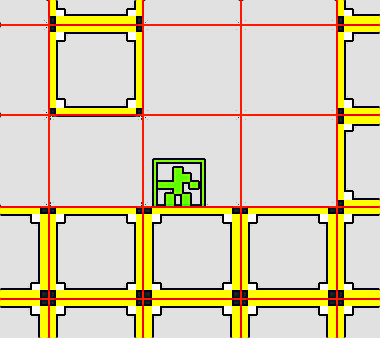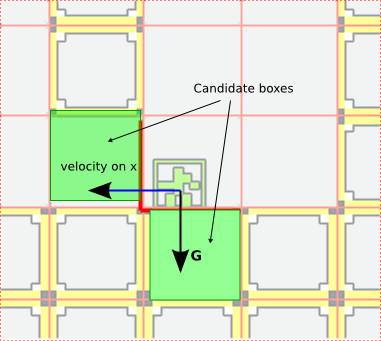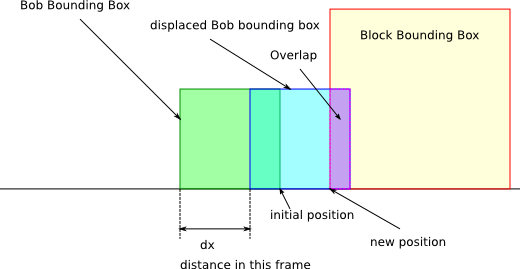Android Games

# Android Game Development with libgdx – Collision Detection, Part 4

This is the fourth part of the libgdx tutorial in which we create a 2d platformer prototype modeled after Star Guard. You can read up on the previous articles if you are interested in how we got here.

Following the tutorial so far we managed to have a tiny world consisting of some blocks, our hero called Bob who can move around in a nice way but the problem is, he

doesn’t have any interaction with the world. If we switch the tile rendering back we would see Bob happily walking and jumping around without the blocks impending him. All the blocks get ignored. This happens because we never check if Bob actually collides with the blocks. Collision detection is nothing more than detecting when two or more objects collide. In our case we need to detect when Bob collides with the blocks. What exactly is being checked is if Bob’s bounding box intersects with the bounding boxes of their respective blocks. In case it does, we have detected a collision. We take note of the objects (Bob and the block(s)) and act accordingly. In our case we need to stop Bob from advancing, falling or jumping, depending with which side of the block Bob collided with.

## The quick and dirty way

The easy and quick way to do it is to iterate through all the blocks in the world and check if the blocks collide with Bob’s current bounding box. This works well in our tiny 10×7 world but if we have a huge world with thousands of blocks, doing the detection every frame becomes impossible without affecting performance.

## A better way

To optimise the above solution we will selectively pick the tiles that are potential candidates for collision with Bob.
By design, the game world consists of blocks whose bounding boxes are axis aligned and their width and height are both 1 unit.
In this case our world looks like the following image (all the blocks/tiles are in unit blocks):

The red squares represent the bounds where the blocks would have been placed if any. The yellow ones are placed blocks.
Now we can pick a simple 2 dimensional array (matrix) for our world and each cell will hold a `Block` or `null` if there is none. This is the map container. We always know where Bob is so it is easy to work out in which cell we are. The easy and lazy way to get the block candidates that Bob can collide with is to pick all the surrounding cells and check if Bob’s current bounding box in overlaps with one of the tiles that has a block.Because we also control Bob’s movement we have access to his direction and movement speed. This narrows our options down even further. For example if Bob is heading left we have the following scenario:The above image gives us 2 candidate cells (tiles) to check if the objects in those cells collide with Bob. Remember that gravity is constantly pulling Bob down so we will always have to check for tiles on the Y axis. Based on the vertical velocity’s sign we know when Bob is jumping or falling. If Bob is jumping, the candidate will be the tile (cell) above him. A negative vertical velocity means that Bob is falling so we pick the tile from underneath him as a candidate. If he is heading left (his velocity is < 0) then we pick the candidate on his left. If he’s heading right (velocity > 0) then we pick the tile to his right. If the horizontal velocity is 0 that means we don’t need to bother with the horizontal candidates. We need to make it optimal because we will be doing this every frame and we will have to do this for every enemy, bullet and whatever collideable entities the game will have.

## What happens upon collision?

This is very simple in our case. Bob’s movement on that axis stops. His velocity on that axis will be set to 0. This can be done only if the 2 axis are checked separately. We will check for the horizontal collision first and if Bob collides, then we stop his horizontal movement.
We do the exact same thing on the vertical (Y) axis. It is simple as that.

## Simulate first and render after

We need to be careful when we check for collision. We humans tend to think before we act. If we are facing a wall, we don’t just walk into it, we see and we estimate the distance and we stop before we hit the wall. Imagine if you were blind. You would need a different sensor than your eye. You would use your arm to reach out and if you feel the wall, you’d stop before you walked into it. We can translate this to Bob, but instead of his arm we will use his bounding box. First we displace his bounding box on the X axis by the distance it would have taken Bob to move according to his velocity and check if the new position would hit the wall (if the bounding box intersects with the block’s bounding box). If yes, then a collision has been detected. Bob might have been some distance away from the wall and in that frame he would have covered the distance to the wall and some more. If that’s the case, we will simply position Bob next to the wall and align his bounding box with the current position. We also set Bob’s speed to 0 on that axis. The following diagram is an attempt to show just what I have described.The green box is where Bob currently stands. The displaced blue box is where Bob should be after this frame. The purple are is how much Bob is into the wall. That is the distance we need to push Bob back so he stands next to the wall. We just set his position next to the wall to achieve this without too much computation. The code for collision detection is actually very simple. It all resides in the `BobController.java`. There are a few other changes too which I should mention prior to the controller. The `World.java` has the following changes:

```public class World {

/** Our player controlled hero **/
Bob bob;
/** A world has a level through which Bob needs to go through **/
Level level;

/** The collision boxes **/
Array<Rectangle> collisionRects = new Array<Rectangle>();

// Getters -----------

public Array<Rectangle> getCollisionRects() {
return collisionRects;
}
public Bob getBob() {
return bob;
}
public Level getLevel() {
return level;
}
/** Return only the blocks that need to be drawn **/
public List<Block> getDrawableBlocks(int width, int height) {
int x = (int)bob.getPosition().x - width;
int y = (int)bob.getPosition().y - height;
if (x < 0) {
x = 0;
}
if (y < 0) {
y = 0;
}
int x2 = x + 2 * width;
int y2 = y + 2 * height;
if (x2 > level.getWidth()) {
x2 = level.getWidth() - 1;
}
if (y2 > level.getHeight()) {
y2 = level.getHeight() - 1;
}

List<Block> blocks = new ArrayList<Block>();
Block block;
for (int col = x; col <= x2; col++) {
for (int row = y; row <= y2; row++) {
block = level.getBlocks()[col][row];
if (block != null) {
}
}
}
return blocks;
}

// --------------------
public World() {
createDemoWorld();
}

private void createDemoWorld() {
bob = new Bob(new Vector2(7, 2));
level = new Level();
}
}```

#09`collisionRects` is just a simple array where I will put the rectangles Bob is colliding with in that particular frame. This is only for debug purposes and to show the boxes on the screen. It can and will be removed from the final game.
#23`getDrawableBlocks(int width, int height)` is the method that returns the list of `Block` objects that are in the camera’s window and will be rendered. This method is just to prepare the application to render huge worlds without performance loss. It’s a very simple algorithm. Get the blocks surrounding Bob within a distance and return those to render. It’s an optimisation.
#61 – Creates the `Level` declared in line #06. It’s good to move out the level from the world as we want our game to have multiple levels. This is the obvious first step. The `Level.java` can be found here.

As I mentioned before, the actual collision detection is in `BobController.java`

```public class BobController {
// ... code omitted ... //
private Array<Block> collidable = new Array<Block>();
// ... code omitted ... //

public void update(float delta) {
processInput();
if (grounded && bob.getState().equals(State.JUMPING)) {
bob.setState(State.IDLE);
}
bob.getAcceleration().y = GRAVITY;
bob.getAcceleration().mul(delta);
checkCollisionWithBlocks(delta);
bob.getVelocity().x *= DAMP;
if (bob.getVelocity().x > MAX_VEL) {
bob.getVelocity().x = MAX_VEL;
}
if (bob.getVelocity().x < -MAX_VEL) {
bob.getVelocity().x = -MAX_VEL;
}
bob.update(delta);
}

private void checkCollisionWithBlocks(float delta) {
bob.getVelocity().mul(delta);
Rectangle bobRect = rectPool.obtain();
bobRect.set(bob.getBounds().x, bob.getBounds().y, bob.getBounds().width, bob.getBounds().height);
int startX, endX;
int startY = (int) bob.getBounds().y;
int endY = (int) (bob.getBounds().y + bob.getBounds().height);
if (bob.getVelocity().x < 0) {
startX = endX = (int) Math.floor(bob.getBounds().x + bob.getVelocity().x);
} else {
startX = endX = (int) Math.floor(bob.getBounds().x + bob.getBounds().width + bob.getVelocity().x);
}
populateCollidableBlocks(startX, startY, endX, endY);
bobRect.x += bob.getVelocity().x;
world.getCollisionRects().clear();
for (Block block : collidable) {
if (block == null) continue;
if (bobRect.overlaps(block.getBounds())) {
bob.getVelocity().x = 0;
break;
}
}
bobRect.x = bob.getPosition().x;
startX = (int) bob.getBounds().x;
endX = (int) (bob.getBounds().x + bob.getBounds().width);
if (bob.getVelocity().y < 0) {
startY = endY = (int) Math.floor(bob.getBounds().y + bob.getVelocity().y);
} else {
startY = endY = (int) Math.floor(bob.getBounds().y + bob.getBounds().height + bob.getVelocity().y);
}
populateCollidableBlocks(startX, startY, endX, endY);
bobRect.y += bob.getVelocity().y;
for (Block block : collidable) {
if (block == null) continue;
if (bobRect.overlaps(block.getBounds())) {
if (bob.getVelocity().y < 0) {
grounded = true;
}
bob.getVelocity().y = 0;
break;
}
}
bobRect.y = bob.getPosition().y;
bob.getBounds().x = bob.getPosition().x;
bob.getBounds().y = bob.getPosition().y;
bob.getVelocity().mul(1 / delta);
}

private void populateCollidableBlocks(int startX, int startY, int endX, int endY) {
collidable.clear();
for (int x = startX; x <= endX; x++) {
for (int y = startY; y <= endY; y++) {
if (x >= 0 && x < world.getLevel().getWidth() && y >=0 && y < world.getLevel().getHeight()) {
}
}
}
}
// ... code omitted ... //
}```

The full source code is on github and I have tried to document it but I will go through the important bits here.

#03 – the `collidable` array will hold each frame the blocks that are the candidates for collision with Bob.
The `update` method is more concise now.
#07 – processing the input as usual and nothing changed there
#08 – #09 – resets Bob’s state if he’s not in the air.
#12 – Bob’s acceleration is transformed to the frame time. This is important as a frame can be very small (usually 1/60 second) and we want to do this conversion just once in a frame.
#13 – compute the velocity in frame time
#14 – is highlighted because this is where the collision detection is happening. I’ll go through that method in a bit.
#15 – #22 – Applies the DAMP to Bob to stop him and makes sure that Bob is not exceeding his maximum velocity.
#25 – the `checkCollisionWithBlocks(float delta)` method which sets Bob’s states, position and other parameters based on his collision or not with the blocks in the level.
#26 – transform velocity to frame time
#27 – #28 – We use a Pool to obtain a Rectangle which is a copy of Bob’s current bounding box. This rectangle will be displaced where bob should be this frame and checked against the candidate blocks.
#29 – #36 – These lines identify the start and end coordinates in the level matrix that are to be checked for collision. The level matrix is just a 2 dimensional array and each cell represents one unit so can hold one block. Check `Level.java`
#31 – The Y coordinate is set since we only look for the horizontal for now.
#32 – checks if Bob is heading left and if so, it identifies the tile to his left. The math is straight forward and I used this approach so if I decide that I need some other measurements for cells, this will still work.
#37 – populates the `collidable` array with the blocks within the range provided. In this case is either the tile on the left or on the right, depending on Bob’s bearing. Also note that if there is no block in that cell, the result is null.
#38 – this is where we displace the copy of Bob’s bounding box. The new position of `bobRec` is where Bob should be in normal circumstances. But only on the X axis.
#39 – remember the collisionRects from the world for debugging? We clear that array now so we can populate it with the rectangles that Bob is colliding with.
#40 – #47 – This is where the actual collision detection on the X axis is happening. We iterate through all the candidate blocks (in our case will be 1) and check if the block’s bounding box intersects Bob’s displaced bounding box. We use the `bobRect.overlaps` method which is part of the Rectangle class in libgdx and returns true if the 2 rectangles overlap. If there is an overlap, we have a collision so we set Bob’s velocity to 0 (line #43 add the rectangle to the `world.collisionRects` and break out of the detection.
#48 – We reset the bounding box’s position because we are moving to check collision on the Y axis disregarding the X.
#49 – #68 – is exactly the same as before but it happens on the Y axis. There is one additional instruction #61 – #63 and that sets the `grounded` state to `true` if a collision was detected when Bob was falling.
#69 – Bob’s rectangle copy is reset
#70 – Bob’s new velocity is being set which will be used to compute Bob’s new position.
#71 – #72 – Bob’s real bounds’ position is updated
#73 – We transform the velocity back to the base measurement units. This is very important.

And that is all for the collision of Bob with the tiles. Of course we will evolve this as more entities are added but for now is as good as it gets. We cheated here a bit as in the diagram I stated that I will place Bob next to the Block when colliding but in the code I completely ignore the replacing. Because the distance is so tiny that we can’t even see it, it’s OK. It can be added, it won’t make much difference. If you decide to add it, make sure sure you set Bob’s position next next to the Block, a tiny bit farther so the overlap function will result
`false`. There is a small addition to the `WorldRenderer.java` too.

```public class WorldRenderer {
// ... code omitted ... //
public void render() {
spriteBatch.begin();
drawBlocks();
drawBob();
spriteBatch.end();
drawCollisionBlocks();
if (debug)
drawDebug();
}

private void drawCollisionBlocks() {
debugRenderer.setProjectionMatrix(cam.combined);
debugRenderer.begin(ShapeType.FilledRectangle);
debugRenderer.setColor(new Color(1, 1, 1, 1));
for (Rectangle rect : world.getCollisionRects()) {
debugRenderer.filledRect(rect.x, rect.y, rect.width, rect.height);
}
debugRenderer.end();
}
// ... code omitted ... //
}```

The addition of the `drawCollisionBlocks()` method which draws a white box wherever the collision is happening. It’s all for your viewing pleasure. The result of the work we put in so far should be similar to this video:

This article should wrap up basic collision detection. Next we will look at extending the world, camera movement, creating enemies, using weapons, adding sound. Please share your ideas what should come first as all are important. The source code for this project can be found here: https://github.com/obviam/star-assault. You need to checkout the branch part4. To check it out with git: `git clone -b part4 git@github.com:obviam/star-assault.git`. You can also download it as a zip file. There is also a nice platformer in the libgdx tests directory. SuperKoalio. It demonstrates a lot of things I have covered so far and it’s much shorter and for the ones with some libgdx experience it is very helpful.

Reference: Android Game Development with libgdx – Collision Detection, Part 4 from our JCG partner Impaler at the Against the Grain blog.

## Want to know how to develop your skillset to become a Java Rockstar?### Impaler

Subscribe
Notify ofThis site uses Akismet to reduce spam. Learn how your comment data is processed.

Inline FeedbacksArtOfPanik
10 years ago

This is an awesome tutorial. By the way, will this collision method work even if the player sprite is bigger than the tile sprite? I’m asking that because I’ve found some issues while working with collisions in Java2D.